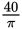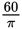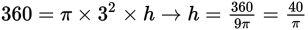# SAT Math Multiple Choice Question 986: Answer and Explanation

### Test Information

Question: 986

6. Milk is poured from a full rectangular container with dimensions 4 inches by 9 inchesby 10 inches into a cylindrical container with a diameter of 6 inches. Assuming allthe milk is transferred without spillage, how many inches high will the milk reachin the cylindrical container?

• A.• B.• C. 24
• D. 30

Getting to the Answer: The volume of milk present is 4 × 9 × = 360 cubic inches. The diameter of the base of the cylindrical container measures 6 inches, so its radius is 3 inches. Substitute this value and the volume of the milk that you found earlier into the formula for finding the volume of a cylinder, and then solve for h:. This means (A) is the correct answer.